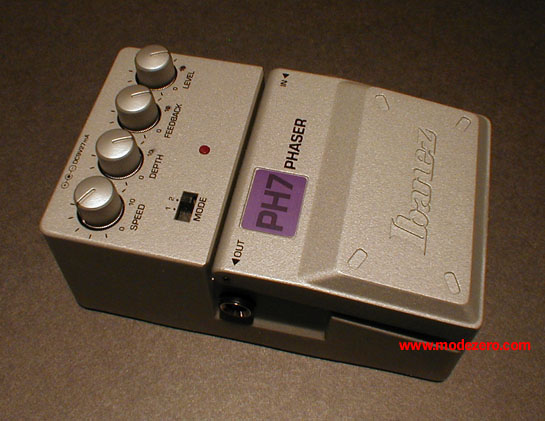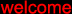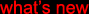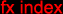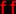(c) 2000-2009

mode zero

ibanez ph7 phaser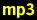guitar, no effectguitar, mode = 1, speed = 6, depth = 10, feedback = 10, level = 10guitar, mode = 2, speed = 6, depth = 10, feedback = 10, level = 10guitar, mode = 2, speed = 9, depth = 2, feedback = 2, level = 10matrix-1000 pad, no effectmatrix-1000 pad, mode = 2, speed = 3, depth = 10, feedback = 10, level = 10matrix-1000 pad, mode = 2, speed = 9, depth = 5, feedback = 0, level = 10drum loop, no effectdrum loop, mode = 2, speed = 5, depth = 2, feedback = 10, level = 10